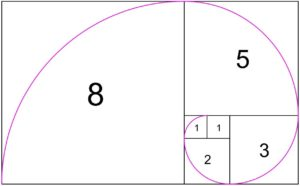# Fibonacci – Golden Sequentials

What fascinated me about this scene while painting my field study was the sense of depth looking down as well as the vastness of the horizon. In re-creating the painting in the studio I felt that the square format would lend itself best to emphasizing the two views. I was satisfied with the initial pass of the studio painting, however I thought that it could be better. I decided to overlay the Fibonacci sequence in Photoshop and that’s where things got interesting.

Based on the square root of five, the Fibonacci sequence is a series of numbers where in every number in it is the sum of the two preceding ones; 1 , 1 , 2 , 3 , 5 , 8 , 13 , 21 , 34 , 55 , 89 , 144. The sequence is also found in the recurring growth pattern found in nature. The proportion of the larger part to the smaller part is the same as the whole, creating a sense of unity. This sequence can be shown in the rectangle geometrically, (Figure i.). In using the overlays, I found that I had hit most of the intersections in the sequence and was able to resolve any issues by applying the ratio.Figure i.

Above: The Fibonacci sequence overlaying my initial pass on the studio painting, turned a 1/4 clockwise, for the four quadrants of the square.

Above at Left: Painting in Photoshop, arc of one square and reversed arc. Note how the intersections of the arc create an Infinity/Vanishing point on the horizon.

Above at Right: Painting in Photoshop, Fibonacci sequence/golden ratio, all rotations visible, plus vanishing point arc.

Note how the space is now divided into logical units. This is similar to using perspective, in that the units of space follow a grid to create depth and a vanishing point(s) through angles. Here the use of curves and intersections divide space that provide a compositional unity. Also, notice that more golden ratio rectangles are formed with the overlays. I could continue with multiple overlays and break the space down further. As the sequence progresses the larger number divided by its preceding smaller number will approach the golden ratio, which is called the “limit” of the Fibonacci sequence. As the sequence of numbers gets greater and greater, the ratios of the larger number divided by the preceding smaller number will get closer and closer to the exact number of the Golden Ratio, 1.618 to 1.